#### 期刊菜单

Advanced Geological Detection for Tunneling Based on Comparative Analysis of Histogram
DOI: 10.12677/HJCE.2020.94032, PDF, HTML, XML, 下载: 373  浏览: 616

Abstract: Based on the construction of several tunnels in Guizhou Province, the relation between image fea-tures of TRT advanced geological prediction and physical truth discovered by excavation was ana-lyzed comparatively, and the TRT image features of several typical adverse geologic phenomena, such as karst cavern, fracture zone, fault, were summarized. According to the features of TRT im-ages, the color histogram contrast method was used to assist the judgment of adverse geologic phenomena from TRT images in the paper. The pick-up and analysis program of color histogram of TRT images was developed based on OpenCV Program. Furthermore, the matching methods be-tween unknown TRT images and the typical TRT images were put forward, and the calculation program was developed to obtain the matching degree which can be used to determine the adverse geologic phenomena reflected by the unknown images. The application of the color histogram con-trast method will promote the quantification and automation of advanced geological prediction, and there was a good achievement in the actual application.

1. 引言

2. 常见不良地质现象TRT特征图像

TRT超前地质预报技术引进国内已有10余年，并在大量隧道工程中进行了应用，不少学者总结了不同不良地质现象的特征图像，但是如前文所述，不同地区与不同地质条件下，相同不良地质现象的特征图像往往亦存在较大差异，因此欲提高超前地质预报的精度，进一步确定工程区域各种不良地质现象的特征图像是必不可少的工作。本文通过跟踪贵州中部地区两条铁路隧道的施工开挖进度，实地对比分析施工揭露的不良地质现象与TRT图像特征的关系，建立了溶洞、破碎带、断层等典型不良地质现象所对应的TRT特征图像库，总结了这些不良地质现象的基本图像特征，为后续超前地质预报计算机自动判译提供依据。

2.1. 破碎带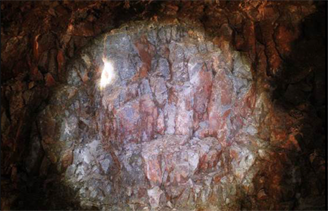Figure 1. The fracture zone located at Section DK320 + 270 of Gaozhaiba Tunnel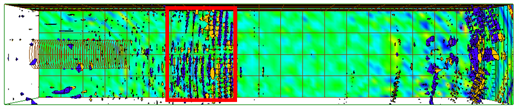(a) 俯视图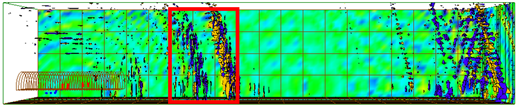(b) 侧视图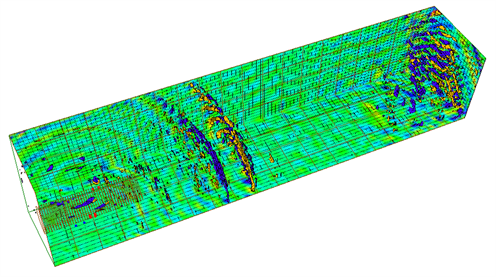(c) 立体图

Figure 2. The TRT figures of Gaozhaiba Tunnel when working face located at Section DK320 + 250

2.2. 溶洞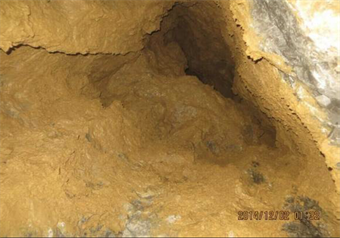Figure 3. TheKarst cavern located at Section DK308 + 120 of Caijiaguan Tunnel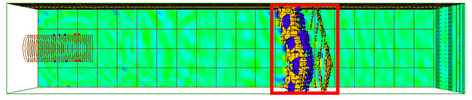(a) 俯视图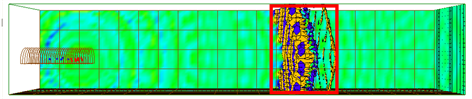(b) 侧视图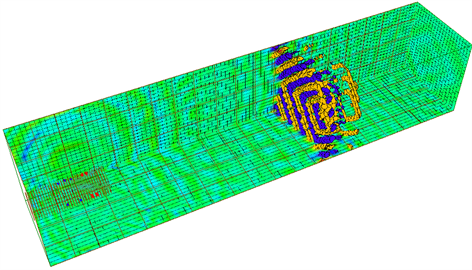(c) 立体图

Figure 4. The TRT figures of Caijiaguan Tunnel when working face located at Section DK308 + 005

2.3. 断层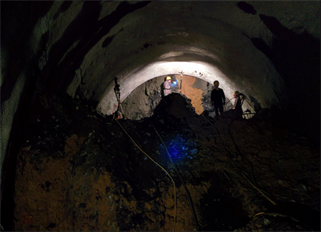Figure 5. The fault located at Section DK37 + 375 of Xiaotianba Tunnel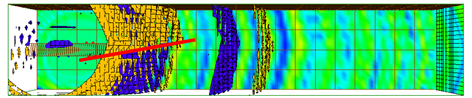(a) 俯视图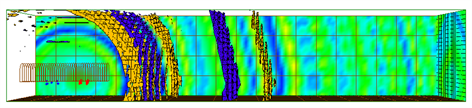(b) 侧视图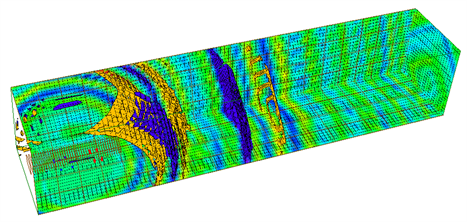(c) 立体图

Figure 6. The TRT figures of Xiaotianba Tunnel when working face located at Section DK37 + 385

3. 颜色直方图对比法

3.1. TRT图像特征分析

TRT图像一大特点是杂乱——没有明显的线条特征，没有能作为基准的兴趣点，而典型的图像识别技术都是基于特征点的识别，如人脸识别、指纹识别等。然而，对TRT图像的识别归根结底是对不良地质现象的识别，而不是对物体本身的识别。不良地质现象不具备稳定的视觉特征，以溶洞为例，溶洞的形态千奇百怪，有管型的、漏斗形的、纺锤形的、硐室形的，发育的方向也是难以捉摸，无法用形状特征予以描述，更不用说纹理以及高层语义特征。因此难以使用传统的基于特征点的图像识别方法对其进行识别。

3.2. 颜色直方图对比法

$H\left(k\right)=\frac{{n}_{k}}{N},k=0,\text{\hspace{0.17em}}1,\text{\hspace{0.17em}}2,\cdots ,L-1$ (1)

3.3. 代码实现

TRT图像识别基于开源代码OpenCV3 (Open Source Computer Vision Library)的算法，对其实例进行了一定程度的修改以适应对TRT图像进行识别的需求，程序基于WIN7 64位旗舰版、Microsoft Visual Studio 2010和OpenCV3，通过C++编写 。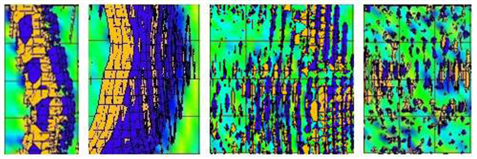Figure 7. Template images of TRT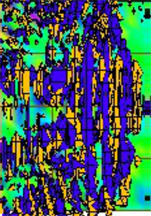Figure 8. The local portion of a TRT image

3.4. 图像对比匹配步骤

TRT图像直方图对比代码实现主要包括读取图片、色彩空间转换、HSV直方图计算、直方图对比四个步骤。

2) 色彩空间转换：对于TRT图像来说，颜色特征并没有自然界场景那么复杂，但由于较为成熟有效的直方图匹配技术都是基于HSV色彩空间的，所以进行直方图对比前要先进行色彩空间的转化，需要注意的是OpenCV读取彩色图像时的通道顺序为蓝绿红(BGR)，而不是我们一般讲的红绿蓝(RGB)；

3) HSV直方图计算：OpenCV中可以使用calcHist( )函数来计算直方图，在使用之前需要初始化直方图实参，选择合适的bin数及取值范围。bin可以翻译为组距，代表着每个特征空间子区段的数目，其数值为从数据中计算出的特征统计量。选择合适数目的bin可以降低直方图维数，提高识别及匹配的效率；

4) 直方图对比：OpenCV中可以使用compareHist( )函数进行直方图对比，此函数的返回值为d(H1, H2)，即两张直方图的距离。

3.5. 对比分析

1) 相关

$d\left({H}_{1},{H}_{2}\right)=\frac{{\sum }_{I}\left({H}_{1}\left(I\right)-\stackrel{¯}{{H}_{1}}\right)\left({H}_{2}\left(I\right)-\stackrel{¯}{{H}_{2}}\right)}{\sqrt{{\sum }_{I}{\left({H}_{1}\left(I\right)-\stackrel{¯}{{H}_{1}}\right)}^{2}{\sum }_{I}{\left({H}_{2}\left(I\right)-\stackrel{¯}{{H}_{2}}\right)}^{2}}}$ (2)

$\stackrel{¯}{{H}_{k}}=\frac{1}{N}\underset{J}{\sum }{H}_{k}\left(J\right)$ (3)

N为第三步设置的bin数。

2) 卡方

$d\left({H}_{1},{H}_{2}\right)=\sum \frac{{\left[{H}_{1}\left(I\right)-{H}_{2}\left(I\right)\right]}^{2}}{{H}_{1}\left(I\right)}$ (4)

3) 直方图相交

$d\left({H}_{1},{H}_{2}\right)=\underset{I}{\sum }\mathrm{min}\left[{H}_{1}\left(I\right),{H}_{2}\left(I\right)\right]$ (5)

4) Bhattacharyya距离(巴氏距离)

$d\left({H}_{1},{H}_{2}\right)=\sqrt{1-\frac{1}{\sqrt{\stackrel{¯}{{H}_{1}}\stackrel{¯}{{H}_{2}}{N}^{2}}}\underset{I}{\sum }\sqrt{{H}_{1}\left(I\right){H}_{2}\left(I\right)}}$ (6)Table 1. Matching degree between Figure 8 and Figure 7

$\stackrel{¯}{{D}_{i}}=\frac{\underset{j=1}{\overset{4}{\sum }}{D}_{ij}}{4}\text{\hspace{0.17em}}\text{\hspace{0.17em}}\text{\hspace{0.17em}}\text{\hspace{0.17em}}\text{\hspace{0.17em}}\left(i=1~4,j=1~4\right)$ (7)

${D}_{ij}=\frac{{d}_{ij}}{\underset{i=1}{\overset{4}{\sum }}{d}_{ij}}\text{\hspace{0.17em}}\text{\hspace{0.17em}}\text{\hspace{0.17em}}\text{\hspace{0.17em}}\left(i=1~4,j=1,\text{\hspace{0.17em}}3\right)$ (8)

${D}_{ij}=\frac{\frac{1}{{d}_{ij}}}{\underset{i=1}{\overset{4}{\sum }}\frac{1}{{d}_{ij}}}\text{\hspace{0.17em}}\text{\hspace{0.17em}}\text{\hspace{0.17em}}\text{\hspace{0.17em}}\left(i=1~4,j=2,\text{\hspace{0.17em}}4\right)$ (9)

${D}_{\text{K}}={D}_{1}+{D}_{2}$ (10)

${D}_{\text{F}}={D}_{3}+{D}_{4}$ (11)

4. 工程应用

4.1. 渝黔铁路中寨隧道

4.2. 织纳铁路小田坝隧道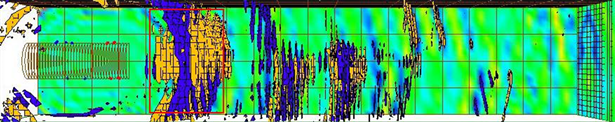Figure 9. Planform of advanced geological prediction in Zhongzhai Tunnel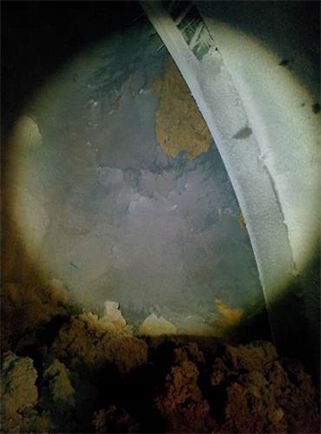Figure 10. The picture of surrounding rock masses in Zhongzhai Tunnel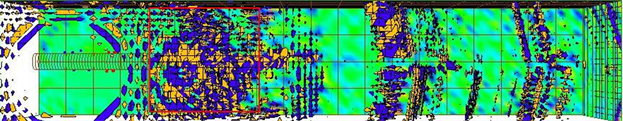Figure 11. Planform of advanced geological prediction in Xiaotianba Tunnel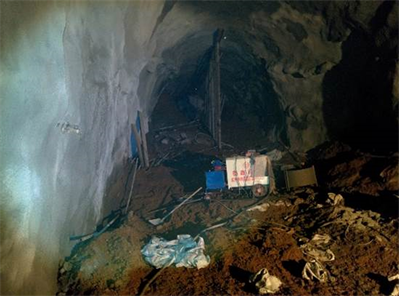Figure 12. The picture of surrounding rock masses in Xiaotianba Tunnel

4.3. 渝黔铁路修文隧道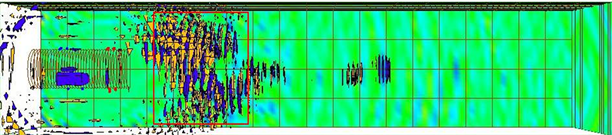Figure 13. Planform of advanced geological prediction in Xiuwen Tunnel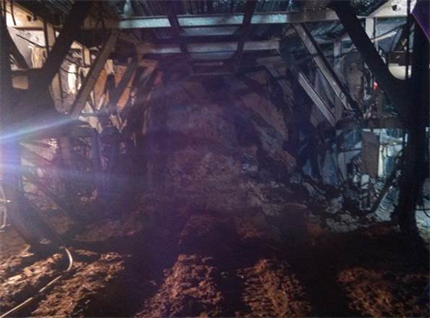Figure 14. The picture of surrounding rock masses in Xiuwen Tunnel

5. 结论

1) 通过跟踪施工开挖进度，实地对比分析施工揭露结果与TRT预报图像特征的关系，总结了溶洞、破碎带、断层等典型不良地质现象所对应的TRT预报图像特征；

2) 基于TRT预报图像的特点，提出采用颜色直方图对比法可以较好地辅助TRT图像的判别，以溶洞和破碎带两种不良地质现象为例，基于OpenCV编制了TRT图像的颜色直方图提取与分析程序；

3) 基于TRT预报特征图像库，提出了未知图像与特征图像的匹配方法，编制了多种匹配方法的计算程序，给出了定量化的匹配度来辅助判断TRT图像反应的不良地质现象。

  钱七虎. 地下工程建设安全面临的挑战与对策[J]. 岩石力学与工程学报, 2012, 31(10): 1945-1956.  李术才, 刘斌, 孙怀凤, 等. 隧道施工超前地质预报研究现状及发展趋势[J]. 岩石力学与工程学报, 2014, 33(6): 1090-1113.  李术才, 聂利超, 刘斌, 等. 多同性源阵列电阻率法隧道超前探测方法与物理模拟试验研究[J]. 地球物理学报, 2015, 58(4): 1434-1446.  张向东, 蔡赫男, 李军. 红庆梁煤矿巷道断层带的超前地质预报[J]. 辽宁工程技术大学学报(自然科学版), 2017, 36(4): 377-380.  姚海波, 苏河修, 梁文广, 等. 基于Google-Earth的地质分析技术在南方某隧道工程地质灾害探测中的应用研究[J]. 土木工程学报, 2017, 50(s2): 167-174.  高峰, 周科平, 周炳仁, 等. 基于TRT技术的矿山井下地质超前预报[J]. 中国安全科学学报, 2014, 24(4): 80-85.  刘新荣, 刘永权, 杨忠平, 等. 基于地质雷达的隧道综合超前预报技术[J]. 岩土工程学报, 2015, 37(s2): 51-56.  刘斌, 李术才, 李建斌, 等. TBM 掘进前方不良地质与岩体参数的综合获取方法[J]. 山东大学学报(工学版), 2016, 46(6): 105-112.  李术才, 苏茂鑫, 薛翊国, 等. 城市地铁跨孔电阻率 CT 超前地质预报方法研究[J]. 岩石力学与工程学报, 2014, 33(5): 913-920.  邱道宏, 李术才, 薛翊国, 等. 基于数字钻进技术和量子遗传–径向基函数神经网络的围岩类别超前识别技术研究[J]. 岩土力学, 2014, 35(7): 2013-2018.  谭信荣, 陈寿根, 王磊, 等. 基于岩溶发育地质模型的隧道地质预测[J]. 地下空间与工程学报, 2015, 11(6): 1641-1647.  曹国侯, 王运生, 李耀华, 等. 三维成像系统在地下工程地质灾害预报中的应用[J]. 地下空间与工程学报, 2014, 10(s1): 1735-1739.  李术才, 石少帅, 李利平, 等. 三峡库区典型岩溶隧道突涌水灾害防治与应用[J]. 岩石力学与工程学报, 2014, 33(9): 1887-1896.  王传武, 李术才, 聂利超, 等. 隧道三维电阻率 E-SCAN 超前探测反演与优化方法研究[J]. 岩土工程学报, 2017, 39(2): 218-227.  李术才, 孙怀凤, 李貅, 等. 隧道瞬变电磁超前预报平行磁场响应探测方法[J]. 岩石力学与工程学报, 2014, 33(7): 1309-1318.  舒森, 胡金星. 隧道岩溶空间探测[J]. 地下空间与工程学报, 2016, 12(5): 1350-1363.  周轮, 李术才, 许振浩, 等. 隧道综合超前地质预报技术及其工程应用[J]. 山东大学学报(工学版), 2017, 47(2): 55-62.  江杰, 黄厚, 钟一和, 等. 岩溶地区地铁一种综合超前地质预报方法[J]. 工程勘察, 2017, 45(6): 69-73.  姚林林, 张世殊, 崔中涛, 等. 双护盾TBM施工综合超前地质预报技术探讨[J]. 地下空间与工程学报, 2019, 15(5): 1549-1556.  任涛, 魏清华, 姜德义, 等. 提高地震区隧道超前地质预报准确性的措施[J]. 地下空间与工程学报, 2016, 12(3): 819-824.  陈礼彪, 刘泉声, 张国华, 等. 非可溶岩隧道突涌灾害预测研究及工程应用[J]. 岩石力学与工程学报, 2014, 33(4): 786-796.  李宝奇, 贺昱曜, 郭元术, 等. 基于DBN的隧道地质超前预报自动解释算法[J]. 长安大学学报(自然科学版), 2017, 37(3): 90-96.  吴回获, 侯小军, 曹小军. 提高TSP超前地质预报准确性的研究与对策[J]. 地下空间与工程学报, 2015, 11(2): 484-488.  江帆. 基于直方图对比TRT图像识别技术的隧道超前地质预报综合判别方法[D]: [硕士学位论文]. 北京: 北京交通大学, 2016.  毛星云, 冷雪飞. OpenCV编程入门[M]. 北京: 电子工业出版社, 2015.